# Google Sheets: Sort by Second or Third character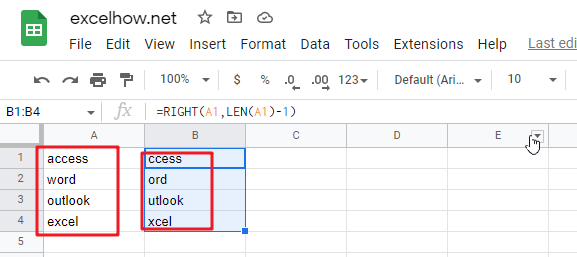This article will talk about how to sort by the second or third character in a cell’s string value in google sheets. You may want to sort the values of strings by the second or last character from the cells in google sheets, how can you do this task quickly? You can use the RIGHT function to create a google sheets formula to solve this problem. The following will show the detailed step-by-step instructions .

## Using the formula to rank by the second character

To sort the test string in the cell by its second character, you can use the RIGHT function and the combination of LEN function to create a new google sheets formula, you could refer to the general formula below.

`=RIGHT(Cell, LEN(Cell)-1)`

This formula will remove the first character of the text string in Cell, then you can use the sort feature to sort this column in google sheets, and then the original column will be sorted as well. Please do it as below.

Suppose you want to sort the A1:A4 range in column A by the second character.

1# Select cell B1 next to cell A1 that contains the data to be sorted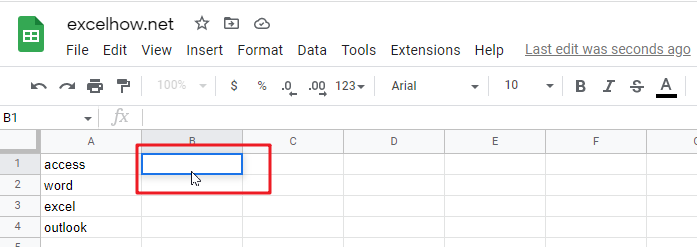2# Enter the following formula in the formula box in cell B1, and then press Enter.

`=right(a1,len(a1)-1)`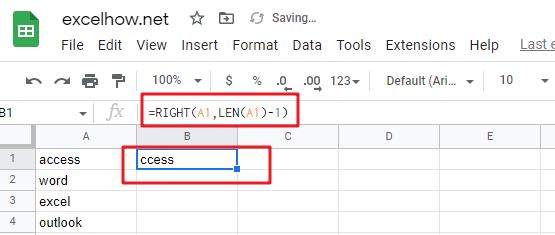3# Select cell B1, then drag the AutoFill handle down to cells B2:B4 to apply this formula. You will see that the first character will be removed.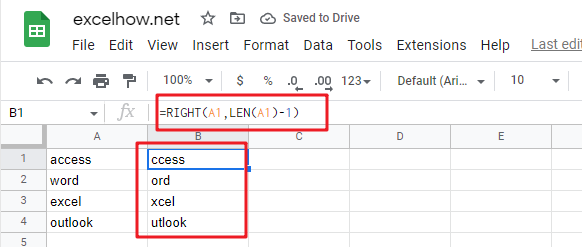4# Select cell B1:B4, click the “Data” menu, and then click the “Sort sheet by column B” submenu under the “Sort sheet” menu.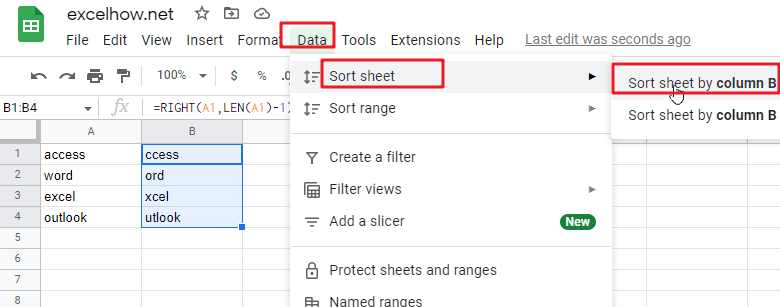5# You would see both column A and column B are sorted by the second character.6# You can now delete column B.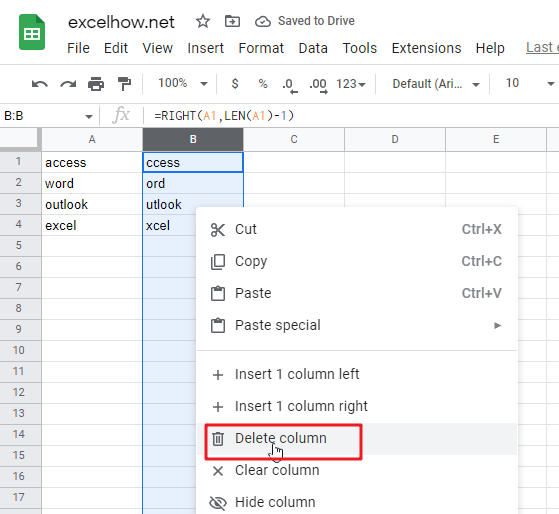If you want to sort by the third character, then you can use the following RIGHT formula, and then follow steps 3-7 to complete the sorting task.

`=RIGHT(Cell, LEN(Cell)-2)`